# Extensions:2.6/Py/Scripts/Nodes/Sverchok

UI location Version Node Editor > Custom nodes > Add nodes 0.2.7 Alexander Nedovzin, Nikita Gorodetskiy, Agustin Jimenez, Linus Yng, Dealga McArdle 2.69 GPL3

File name __init__.py http://github.com/nortikin/sverchok 2014-01-12

## What is Sverchok

• Sverchok IS a tool for generating parametric geometry. We plan on supporting these features until version 2.0.
• Sverchok IS NOT a complete node-based redesign of Blender's UI. It does not support node based particles, lighting etc.
• Sverchok does not incorporate elements of pynodes' framework spector, because we are focused on a different aim - generating geometry. We are interested in developing Sverchok to work cooperatively with other node systems such as Lukas Toene's node based particle systems.
• Main developing line: MATH + MESH + SPLINES + NURBS.

## Screenshots

Sverchok is a tool that allows you to generate parametric and procedural forms and geometry with nodes, without having to know any programming or scripting languages.

I don't know why these gif images animations do not fit to the thumbnails. They are just full-sized

## How to Use

### Installation

Install the add-on by opening User Preferences and selecting "Install from file..." in the Add-ons tab. Check the checkbox on the right.

### Data structure and sockets (slots on nodes)

FOR DETAILED INSTRUCTIONS ON DATA LOOK HERE: Data structure of sverchok

Sverchok has specific data structure:

```  What is data in Sverchok:

[[(x0,y0,z0),(x1,y1,z1),(x2,y2,z2)][...     ]...]  that is how it looks 0,1,2,3
0 [                                               ]  socket's container list - essential
1  [object 0                        ][object 1]...   object, matrix, as one list, as many objects so many lists here
2   (vert 0  ),(vert 1  ),(vert 2  )  (...   )       lists
3    x0,y0,z0   x1,y1,z1   x2,y2,z2                  integers, floats
```

Sockets are:

1. Vertices socket represent vectors, that define mesh (vertices are vectors and vice versa):
```Vertices tree:

[[(x,y,z)]]
0 [ object,... ]
1 obect = [ v... ]
2 v = (x,y,z)
3 x,y,z = float,float,float
```
1. Edges/Polygons/Data socket. cat transfer any data, simply used for create lists, floats, and used as lists of edges and polygons. in viewer it define itself, which kind of data you connect, if in list two elements - it is edges (connect integers), if in list more than two - it is polygons and automatically it draws polygons in 3d view or bake it to blender object with mesh.
```Edges/polygons tree:

[[(n...)]]
0 [ object ]
1 object = [pol...]
2 pol = [n,n,n,n,n]
3 n = integer
```
1. Matrixes consists of 4 lines at 4 floats, it defines rotation, scale, translation. It is mathimatically usefull to operate with matrixes. Only one matrix for one object. To draw several objects with the same mesh, you have to connect several matrixes, each of it defines object's location, rotation, scale.
```Matrixes tree:

[[(x,y,z,w)]]
0 [ matrix ]
1 matrix = (a,b,c,d)
2 a,b,c,d = (x,y,z,w)...
3 x,y,z,w = float
```

Nikitron 17:59, 22 April 2014 (CEST)

# Nodes

### Basic

1.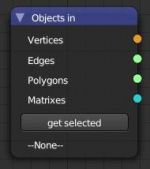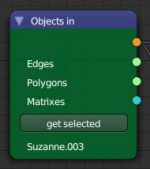Objects in - take data from object
2. Bake all - bakes all geometry on fly - under development
3.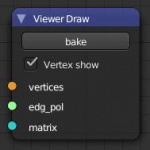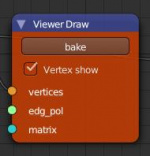Viewer draw - draw data in OpenGL and bakes viewed object
4.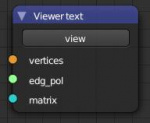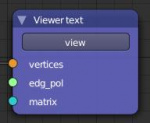Viewer text - show data in "text editor" in "Sverchok text" file
5. Viewer INDX - show vertices indeces in 3D. lesson howto index
6.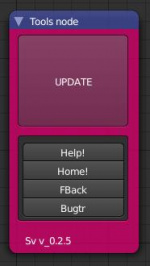Tools - update all nodes, help, home site, feedback
7. Wifi in - node to take sockets' values without wires on layout. Needed personal naming in special field to be the same as in output wifi. Work in pare.
8. Wifi out - node to give sockets' values without wires on layout. Needed personal naming in special field to be the same as in input wifi. Work in pare.
9. Text in - takes data from text file. Lesson_1, lesson_2
10. Text out - makes data writed to text in some format - cvs, sverchok or other type. Than you can export it to some format
11. Frame info - takes current frame, start, end frames
12. Note - lets you leave notes on layout note node showed

Nikitron 17:59, 22 April 2014 (CEST)

### List

1.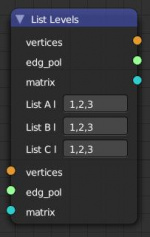List Levels - leave selected levels and delete other levels of nasted lists
2.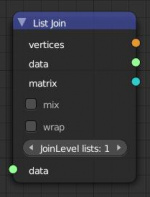List Join - joins some lists in many ways
3.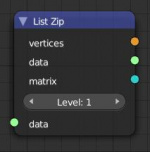List Zip - join several objects from one socket, but can join lists
4.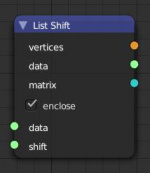List Shift - shift items to some value usefull in polygons definition
5.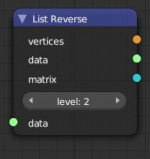List Reverse - make list 1,2,3,4,5,6 reversed as 6,5,4,3,2,1
6.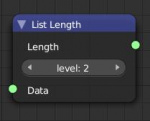List Length - length of selected list level
7.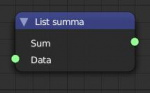List Sum - Sum of all items in list (floats and int)
8.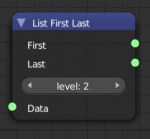List First&Last - get first and last item in list on selected data level
9. List Item - pick item
10. List Repeater - repeat some user-defined level's list some user-defined times
11. List Math - operates with lists - takes maximum value, minimum value and avarage value
12. List Flip - flip some level of list that row becomes column and vise versa
14. List Sort - sort list
15. List Shuffle - randomise list
16. List Match - making one list match in length for another and vice versa. One ca be shorten, cycled prolonged or repeated prolonged in different levels
17. Socket convert - temporery solution till we have adaptive sockets universal

Nikitron 17:59, 22 April 2014 (CEST)

### Number

1.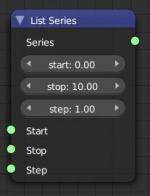Series - makes range of floats
2. Range - the same, but diapason devided by division's count
3. List input - creates list from exact values as many as needed
4.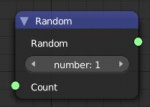Random - makes so many rendom floats as you tell him. Random from 0 to 1
5.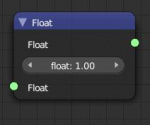Float - make float value
6.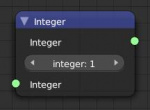Int - make integer value
7.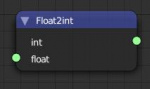Float 2 Int - make integer from float
8.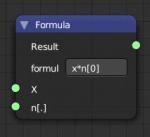Formula - calculate values by some expressions defined by user (+, -, /, *, min, max, sin, cos, etc.)
9. Math - math functions in one node
10.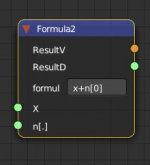Formula2 - support vector*vector, define hierarhy and calculate respectfully to it. Supporting additionally to formula:
```        acos()
acosh()
asin()
asinh()
atan()
atan2()
atanh()
ceil()
copysign()
cos()
cosh()
degrees()
e
erf()
erfc()
exp()
expm1()
fabs()
factorial()
floor()
fmod()
frexp()
fsum()
gamma()
hypot()
isfinite()
isinf()
isnan()
ldexp()
lgamma()
log()
log10()
log1p()
log2()
modf()
pi
pow()
sin()
sinh()
sqrt()
tan()
tanh()
trunc()
==
!=
<, >
for, in, if, else
[]
```

and output to vector. Moreover, you can define lists with formula, i.e. 0,1,2,3,4,5 for series or (1,2,3),(1,2,3) for vertices Nikitron 17:59, 22 April 2014 (CEST)

### Generators

1.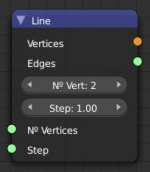Line - fully makes line - vertices and edges, can take series of steps
2.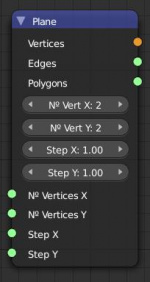Plane - fully makes plane grid with steps and number of dots, as line do
3. Box - could be subdivided on X,Y,Z
4. Cyrcle - could be segmented or chorded
5. Cylinder - subdivisions in height
6. Sphere - usual Sphere
7. Hilbert - Гильбертово пространство, квадрат всегда
8. Hilbert Image - hilbert from image
9. Voronoi - makes voronoi 2D field from vectors
10. Image - mesh defined by image
11. Random Vector - makes unit normal vectors in random values
12. Scripted Node - you making script and node appears. details lesson how to make your custom script, lesson how to bind your script to script node
Here are the current implementation rules for node-loadable scripts for Scripted Node:
We have simplifyed syntax - no need if than wxpression with {0}... See templates
• the main working function must be called sv_main
• function def must have input variables and defaults. It could be def sv_main(veriable=12) for integers/floats defaults or def sv_main(veriable=[]) for lists and series of floats/integers
• must have in_sockets and out_sockets , as shown in examples and return them
• you can create three types of in/out sockets:
1. 'v' - Vectors socket
2. 's' - edges/polygons/numbers/else socket
3. 'm' - matrices socket
it looks like:
in_sockets = [
['s', 'Vertices', data],
['s', 'Step', step],
]
• you can define def sv_main(**your_veriables_here**): and press CTRL+I in text area to automake veriables in in_sockets list. All for your convenient.
Please, use templates in node (popup and button)

Nikitron 23:48, 3 May 2014 (CEST)

### Vectors

Vector - is list of three floats (x,y,z), defining 3D situation of one dot

Matrix - here you can find links to educate what is matrix - is three vectors responsible for Location, Scale, Rotation. We define that or change or multiply.

1.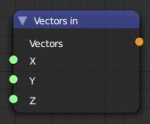Vector in - make Vector
2.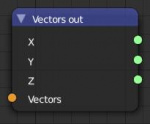Vector out - destruct vector to XYZ
3.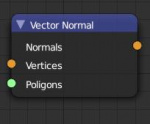Vector normal - gives normal of vector from polygoons and vertices
4.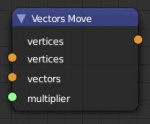Vector Move - moves vectors to another verctors
5. Vector Math - Basic Vector functions in one node. Add, Sub, Normal, Distance, Projection, Cross Product, etc
6. Matrix apply - apply matrix (locatin, rotation, scale) to vertices, similar to ctrl+A in blender
7. Vector Drop - makes polygons turned to floor, as they lay on floor. usefull for farthere usage in bezier curve 2D operations and making easy DWG layout to plot or something else. Here we have usage of Vector Drop
8. Vector X Doubles - remove doubled vertices (twiced node with other in modifiers - need to be removed doubled itself. that irony)
9. Vector Evaluate - find points from one point to other - can have minus values and more than 1
10. Vertices Sort - sorting vertices at one direction or another
11. Matrix Apply - apply Tra/Rot/Sca values to vertices as alt+A
12.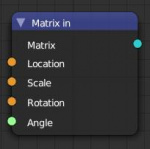Matrix in - generate matrix
13.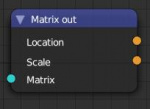Matrix out - destroy matrix to location and scale
14.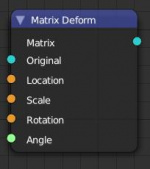Matrix Deform - define scale, rotate or translate matrixes. It is able to rotate matrix with two vectors: Put initial vector to Rotation Axis vector socket and second to Angle. It will rotate on difference between angles Rot-Ang. It is showed in Spider example file on official site's examples.
15. Shear Matrix - makes matrix to shear as shift up to one side, bottom to another, for example lesson how to shear
16. Matrix interpolation - defines "mutations" for middle-deformed matrixes.

### Modifier

1.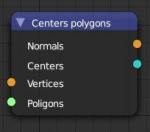Centers Polygons - find polygon's centers
2.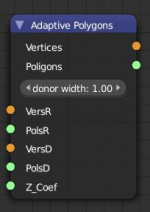Adaptive polygons - makes donor object spreads on recipient object adaptively to each polygon's corners. Donor has to be square on base, and base square has to locate on local zero Z-axis. Lesson how to adaptive polygons
3. Adaptive Edges - replace some edge of recipient with donor's edges.
4.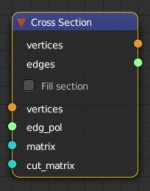Cross Section old - makes section between object and plane. Plane - it is matrix' XY plane, so many matrixes makes many sections
5. Bisect new - makes crossection with more functions and quicker Here we have how to bisect (cross section)
6. Solidify - makes mesh extruded
7. Wireframe - makes wireframed mesh
8. Line Connection - by edges/polygons (not fully ended) Lesson how to line connection
9. Delanuary 2D - makes polygons from vectors
10. Voronoi - makes voronoi edged mesh in floor, 2D frm defined points
11. Polygon Boom - separates polygons to objects and dropping them to zero point - here we have polygon boom usage
12. Polygons to Edges - converts polygons to edges Lesson how to polygon to edge
13. Mesh Join - join several meshes with preserve of vertex indexes in polygons/edges
14. Mesh Remove Doubles - removes doubled vercites and polygons and edges
15. Delete Loose - deleting free vertices left from edges/pols, that use some vertices﻿
16. Convex Hull - as Delaunay node makes triangles on vertices, but in 3D
17. Intersect edges - finding intersections of edges

### Analiser

1.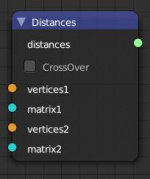Distances - find distances between matrixes or vertices
2. Area - area of polygons and theyr's sum
3. KDTree vertices - finding closest point to other points list
4. KDTree edges - creating edges based on distance between vertices